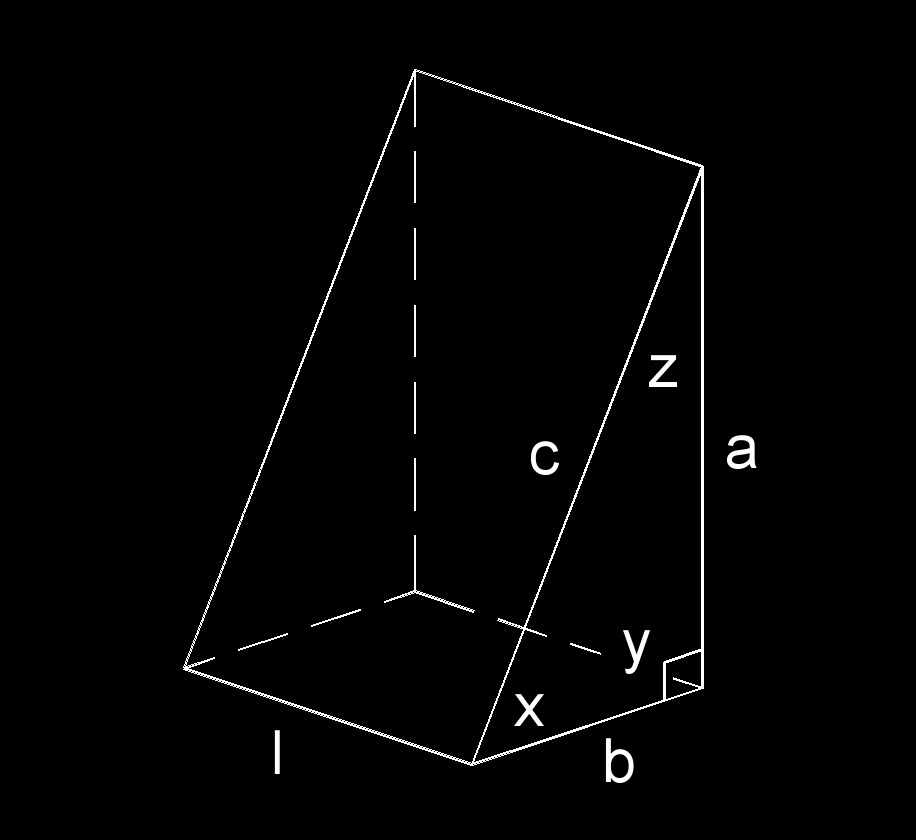# Right Triangle Wedge

Written by Jerry Ratzlaff on . Posted in Solid Geometry

•Right triangle wedge (a three-dimensional figure) has two right triangle ends and three rectangle sides.
• 3 rectangles
• 2 right triangles
• 1 base
• 9 edges
• 2 ends
• 2 sides
• 6 vertexs

### Angle of a Right Triangle Wedge formula

$$\large{ x = arccos \; \left( \frac{b^2+c^2-a^2}{2\;b\;c} \right) }$$

$$\large{ z = 90° - x }$$

Where:

$$\large{ x, z }$$ = angle

$$\large{ a, b, c }$$ = edge

### Hypotenuse of a Right Triangle formula

$$\large{ c = \sqrt{a^2+b^2} }$$

Where:

$$\large{ c }$$ = hypotenuse (H)

$$\large{ a, b }$$ = edge

### Surface area of a Right Triangle Wedge formula

$$\large{ A_s = a\;b+l \; \left( a+b+c \right) }$$

Where:

$$\large{ A_s }$$ = surface area

$$\large{ a, b, c }$$ = edge

$$\large{ l }$$ = length

### Volume of a Right Triangle Wedge formula

$$\large{ V = \frac{l\;a\;b}{2} }$$

Where:

$$\large{ V }$$ = volume

$$\large{ l }$$ = length

$$\large{ a, b, c }$$ = edge